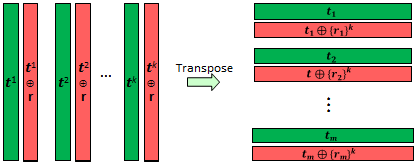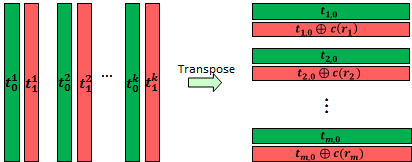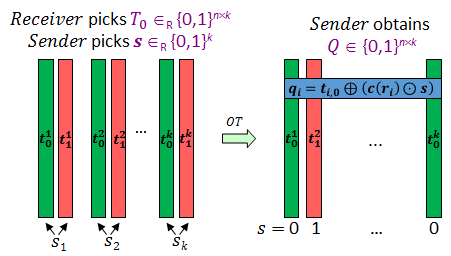## Improved OT Extension for Transferring Short Secrets

CRYPTO 2013 [pdf] [bibtex]

### Prerequisite:

This summary expects the reader to have a basic understanding of the IKNP03 protocol.

# Introduction

KK describe an generalization and optimization to IKNP03 protocol for ⚠ {$OT$} extension in the semi-honest setting. The protocol provides a ⚠ {$O(\log k)$} performance improvement over the IKNP 1-out-of-2 ⚠ {$OT$} protocol and generalizes it to a 1-out-of-⚠ {$n$} ⚠ {$OT$} with the same asymptotic improvement over the state of the art 1-out-of-⚠ {$n$} ⚠ {$OT$}. The protocol is also applicable in the multi-party setting unlike the original IKNP version. In concrete terms, this yields a 2-3 factor performance improvement for the 1-out-of-2 ⚠ {$OT$} protocol.

The main observation made by KK is that in the IKNP protocol, the ⚠ {$Receiver$}'s selection vector ⚠ {$r$} is reused on each of the ⚠ {$k$} initial ⚠ {$OT$}s. This can be seen below on the left.On each one of the (red/green) Column pairs, a 1-out-of-2 ⚠ {$OT$} is performed in the IKNP protocol. If you take the transpose of these columns, each red row encoding is ⚠ {$t_j\oplus \{1\}^k$} or ⚠ {$t_j\oplus \{0\}^k$} based upon the bit ⚠ {$r_j$}. Inspired by the observation that these repetitious codes (⚠ {$\{1\}^k$} and ⚠ {$\{0\}^k$}) dictate the receivers selection, what if the codes were vectors of ⚠ {$\{0,1\}^k$}. Would this enable to protocol to generalize to a 1-out-of-⚠ {$n$} ⚠ {$OT$}?

To accommodate a 1-out-of-⚠ {$n$} ⚠ {$OT$} protocol, the IKNP ⚠ {$Receiver$} selection vector ⚠ {$r\in \{0,1\}^m$} will need to be expanded to ⚠ {$r\in \{0,1,...,n-1\}^m$} to denote the ⚠ {$Receiver$}'s 1-out-of-⚠ {$n$} selection on all ⚠ {$m$} ⚠ {$OT$}s.

To securely achieve this result, the new ⚠ {$\{0,1\}^k$} vector codes will need to have a relative distance of ⚠ {$\frac{1}{2}$} between each other. That is, each code must be different enough from the others to maintain the security. The Walsh-Hadamard codes have this exact property. We will denote the Walsh-Hadamard encoding of ⚠ {$r_j$} as ⚠ {$c(r_j)$}.

# 1-out-of-n Protocol

Let the ⚠ {$Receiver$} generate ⚠ {$T_0$} as a ⚠ {$m \times k$} random bit matrix, shown below as the green vectors. Let ⚠ {$T_1$} be the matrix whose ⚠ {$j^{th}$} row is the ⚠ {$j^{th}$} row of ⚠ {$T_0$} XOR ⚠ {$c(r_j)$}, as seen below by the red vectors. Note: ⚠ {$k$} is the security parameter.where ⚠ {$t_{j,0}$} is the ⚠ {$j^{th}$} row of ⚠ {$T_0$}, and ⚠ {$t_x^i$} is the ⚠ {$i^{th}$} column of ⚠ {$T_x$}

The protocol now performs ⚠ {$k$} 1-out-of-2 ⚠ {$OT$}s on the ⚠ {$k$} columns of the two matrices ⚠ {$T_0$} and ⚠ {$T_1$}, where the ⚠ {$Receiver$} acts as the sender. Note: these ⚠ {$OT$}s are the same as the IKNP protocol except that the rows of ⚠ {$T_1$} are ⚠ {$t_{j,0}$}⚠ {$\oplus c(r_j)$} instead of ⚠ {$t_{j,0}$}⚠ {$\oplus \{r_j\}^k$}.

As before in IKNP, the ⚠ {$Sender$} will randomly select between these two (red/green) columns for each of the ⚠ {$k$} pairs. Let ⚠ {$s\in \{0,1\}^k$} be the vector denoting this random selection and let ⚠ {$Q$} be the matrix denoting the collection of these ⚠ {$k$} ⚠ {$OT$}s when they are stored column wise. That is ⚠ {$q^i=t^i_{s_i}$}As shown in blue, each row of ⚠ {$Q$} (denoted as ⚠ {$q_i$}) will be the corresponding row in ⚠ {$T_0$} XORed with the Walsh-Hadamard code for ⚠ {$r_i$} bitwise ANDed with ⚠ {$s$}. The main take away here is that each row of ⚠ {$Q$} has 1-out-of-⚠ {$n$} possible values if you fix ⚠ {$T_0$} and ⚠ {$s$}. In particular, this 1-out-of-⚠ {$n$} decision is made by the ⚠ {$Receiver$} and is unknown to the ⚠ {$Sender$}.

The initialization phase has now completed and the real 1-out-of-⚠ {$n$} ⚠ {$OT$}s can now be performed.

The ⚠ {$Sender$} will send the ⚠ {$Receiver$} an one time pad encryption of each of the ⚠ {$n$} messages for each of the 1-out-of-⚠ {$n$} ⚠ {$OT$}s. The ⚠ {$i^{th}$} messages in this ⚠ {$OT$} will be encrypted with the hash, ⚠ {$H(j,q_j\oplus ( c(i)\odot s ))$} as a one time pad mask. Based upon the ⚠ {$receiver$}'s choice of ⚠ {$r_j$}, exactly one of these messages will be equivalent to ⚠ {$H(j,$}⚠ {$t_{j,0}$}⚠ {$)$}. That is ⚠ {$q_j\equiv$}⚠ {$t_{j,0}$}⚠ {$\oplus (c(r_j)\odot s)$} will cancel with ⚠ {$( c(i)\odot s )$} leaving ⚠ {$t_{j,0}$} when ⚠ {$i=r_j$}.

Put algorithmically,
For each ⚠ {$j$} in the ⚠ {$m$} ⚠ {$OT$}s:

For each ⚠ {$i$} in the ⚠ {$n$} messages of the ⚠ {$j^{th}$} ⚠ {$OT$}:
The ⚠ {$Sender$} sends the ⚠ {$Receiver$}: ⚠ {$y_{j,i}=M_{j,i}\oplus H(j,q_j\oplus ( c(i)\odot s ))$}

Where ⚠ {$M_{j,i}$} is the ⚠ {$i^{th}$} message of the ⚠ {$j^{th}$} ⚠ {$OT$}.

The ⚠ {$Receiver$} will then decrypt the ⚠ {$y_{j,r_j}$} message by re-XORing ⚠ {$y_{j,r_j}$} with ⚠ {$H(j,$}⚠ {$t_{j,0}$}⚠ {$)$}. This results in ⚠ {$M_{j,r_j}$}, which is precisely the ⚠ {$Receiver$}'s desired message on the ⚠ {$j^{th}$} ⚠ {$OT$}. All of the other message encryptions of the ⚠ {$j^{th}$} ⚠ {$OT$} will remain pseudo random because the ⚠ {$Receiver$} does not know the random bit vector ⚠ {$s$} that the ⚠ {$Sender$} picked.

In sum, ⚠ {$m$} instances of a 1-out-of-⚠ {$n$} ⚠ {$OT$} can be obtained by ⚠ {$k$} instances of a 1-out-of-2 ⚠ {$OT$} plus at most ⚠ {$mn$} calls to a random oracle by both parties. Since ⚠ {$k$} is the security parameter this can yeild a significant improvement when ⚠ {$m$} becomes large.

# 1-out-of-2 Optimization

Let ⚠ {$n\choose 1$}-⚠ {$OT_{l}^{m}$} denote ⚠ {$m$} instances of a 1-out-of-⚠ {$n$} ⚠ {$OT$} each of length ⚠ {$l$}.

From an observation not shown here, ⚠ {$\log n$} instances of a ⚠ {$2\choose 1$}-⚠ {$OT$} can be obtained from a single instance of ⚠ {$n\choose 1$}-⚠ {$OT$} of slightly longer message length. Put precisely, a ⚠ {$2\choose1$}-⚠ {$OT_{l}^m$} has the same exact cost as a ⚠ {$n\choose 1$}-⚠ {$OT_{l\log n}^{m/\log n}$}. This hints that the generalization KK describe can also be used to further optimize the 1-out-of-2 variant.

With the use of this observation, ⚠ {$m$} instances of a 1-out-of-2 ⚠ {$OT$} can be obtained with the additional asymptotic cost of ⚠ {$O(mk/\log(k/l))$}, where ⚠ {$k$} is the security parameter. This contrasts with the IKNP cost of ⚠ {$O(m(k+l))$}. In the important case when ⚠ {$l=1$}, we obtain a logarithmic improvement over IKNP.

## Random Oracle

Similar to the IKNP protocol, a ⚠ {$Code$}-⚠ {$Correlation$} ⚠ {$Robust$} random oracle is required to ensure a semi-honest ⚠ {$Receiver$} cannot learn ⚠ {$s$} and therefore all of ⚠ {$M$}. ⚠ {$Code$}-⚠ {$Correlation$} ⚠ {$Robustness$} is a property of a random oracle ensuring that no information about the input is leaked when the input are known values XORed/ANDed with an unknown value. Even if polynomial many queries with different know values are made. Specifically, the joint distribution of

⚠ {$\{t_1,t_2,...,t_n\}$} , ⚠ {$\{c_1,c_2,...,c_n\}$} and ⚠ {$H(t_j\oplus (c_{j'}\odot s)),H(t_l\oplus (c_{l'}\odot s)),...,H(t_n\oplus (c_{n'}\odot s))$}

must be pseudo random, where ⚠ {$s$} is unknown and ⚠ {$H$} is queried a polynomial number of times with respect to the security parameter. This is a requirement because the ⚠ {$Receiver$} knows all of these ⚠ {$t_i$} and ⚠ {$c_i$} values. i.e. ⚠ {$t_{i,0}$} and ⚠ {$c(r_i)$}. If the correlations between the inputs and the hash values are not pseudo random, the ⚠ {$Receiver$} could learn ⚠ {$s$}, and thereby all of ⚠ {$M$}.

# Summary

In summary, this protocol reduces ⚠ {$m$} instances of a 1-out-of-⚠ {$n$} ⚠ {$OT$} to a small security parameter number of 1-out-of-2 ⚠ {$OT$}s. In practice ⚠ {$m$} can be several orders of magnitude larger than the security parameter ⚠ {$k$}. For example ⚠ {$m=30000, k=256$}. This yields a significant performance increase over a na�ve implementation of ⚠ {$m$} instances of a 1-out-of-⚠ {$n$} ⚠ {$OT$}. At the time this paper was published, the protocol results in a logarithmic improvement over the state of the art, which translates to an approximate speed up of 5 fold.

In addition, this 1-out-of-⚠ {$n$} protocol yields a performance improvement for the 1-out-of-2 variant when they are implemented by a black box use of this 1-out-of-⚠ {$n$} protocol. Beyond these improvement, KK describe another optimization by using a pseudo random generator instead of the completely random matrix ⚠ {$T_0$}.